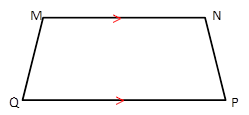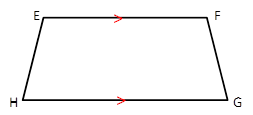Chapter 4.4, Problem 27EElementary Geometry For College St...

7th Edition
Alexander + 2 others
ISBN: 9781337614085

Solutions

Chapter
SectionElementary Geometry For College St...

7th Edition
Alexander + 2 others
ISBN: 9781337614085
Textbook Problem

EFGH and MNPQ are isosceles trapezoids. Are these trapezoids congruent ifa) E F ¯ ≅ M N ¯             a n d             H G ¯ ≅ Q P ¯ ? b) E F ¯ ≅ M N ¯     ,       H G ¯ ≅ Q P ¯ ,               a n d             E H ¯ ≅ M Q ¯ ?To determine

To Find:

Whether the given trapezoids are congruent.

Explanation

The given trapezoids EFGH and MNPQ are

We know that for two trapezoids to be congruent, the legs of the trapezoids needs to be congruent.

Also, for an isosceles trapezoid EF¯||HG¯ and EH¯FG¯

To determine

To Find:

Whether the given trapezoids are congruent.

Still sussing out bartleby?

Check out a sample textbook solution.

See a sample solution

The Solution to Your Study Problems

Bartleby provides explanations to thousands of textbook problems written by our experts, many with advanced degrees!

Get Started

Prove that limx21x=12.

Single Variable Calculus: Early Transcendentals, Volume I

Expand each expression in Exercises 122. (x32x2+4)(3x2x+2)

Finite Mathematics and Applied Calculus (MindTap Course List)

Fill in each blank: 6lb9oz=oz

Elementary Technical Mathematics

In problems 1-4, use or to indicate whether the given object is an element of the given set. 3.

Mathematical Applications for the Management, Life, and Social Sciences

Divide the following fractions and reduce to lowest terms. 23.

Contemporary Mathematics for Business & Consumers